# Generating Polar and Parametric Plots in Wolfram|Alpha

August 3, 2011 —Comments Off

In my last blog post on plotting functionality in Wolfram|Alpha, we looked at 2D and 3D Cartesian plotting. In this post, we will look at 2D polar and parametric plotting.

For those of you unfamiliar with polar plots, a point on a plane in polar coordinates is located by determining an angle θ and a radius r. For example, the Cartesian point (x, y) = (1, 1) has the polar coordinates (r, θ) = (√2,π/4). The following diagram illustrates the relationship between Cartesian and polar plots.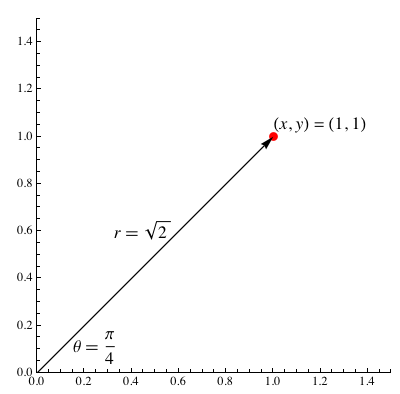To generate a polar plot, we need to specify a function that, given an angle θ, returns a radius r that is a function r(θ). Making a polar plot in Wolfram|Alpha is very easy; for example, we can plot Archimedes’ spiral.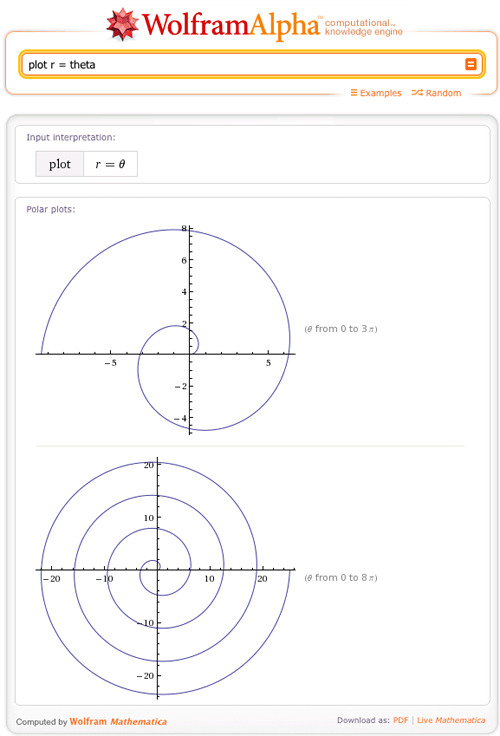Or we can get a little fancier and plot a polar rose with eight petals.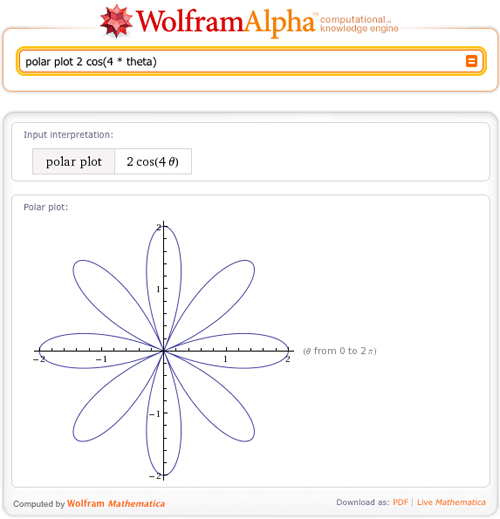Want to know how to graph this in Mathematica? We can easily extract the Mathematica code for this plot right from Wolfram|Alpha. By clicking the dog-ear in the bottom left of the images and then “Copyable plaintext”, you can see the Mathematica code used to generate the plots.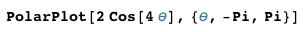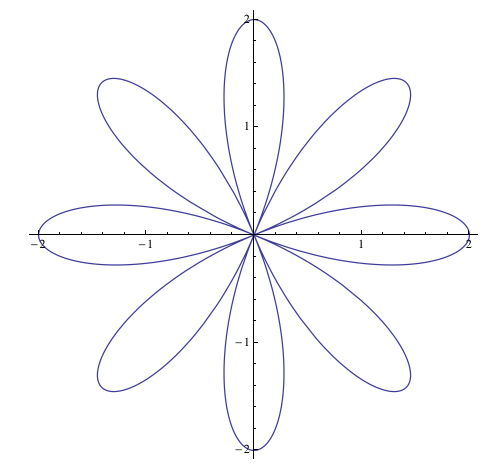Here are some other awesome polar plots:

The plot of a lemniscate: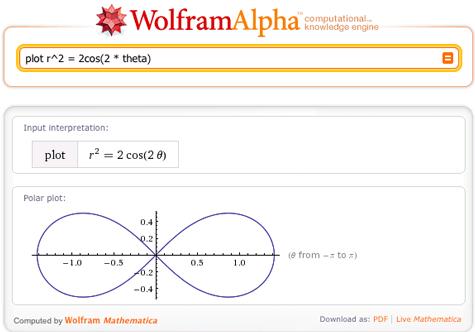And a lituus: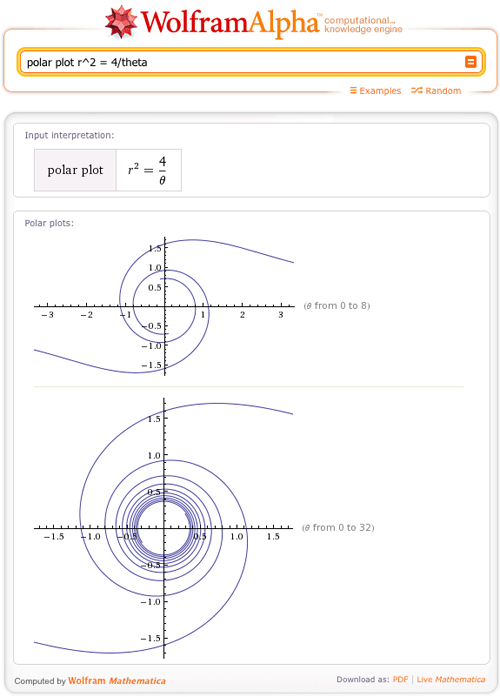And a cochleoid: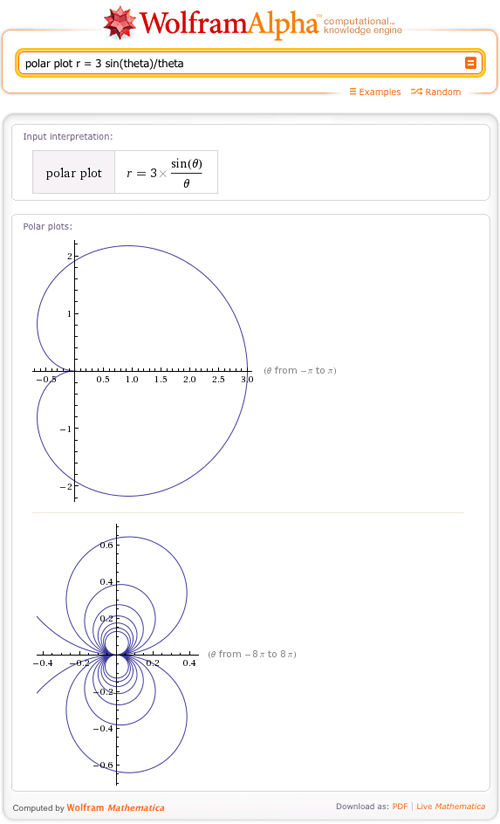Wolfram|Alpha can also handle more complicated inputs, like r(θ) = exp(cos(θ) – 2 cos(4θ) + sin (θ/12)^5: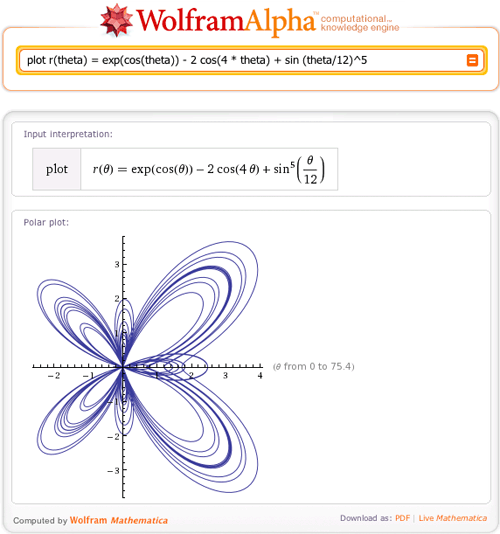Now that you have seen some great examples of polar plots, let’s move on to parametric plots.

What is the difference between a polar and parametric plot? Parametric coordinates specify points (x,y) in 2D with two functions, (x,y) = (f(t), g(t)) for a parameter t. Here are some examples of 2D parametric plots to try in Wolfram|Alpha.

Try to make a parametric plot of (x(t), y(t)) = (1-t,t2).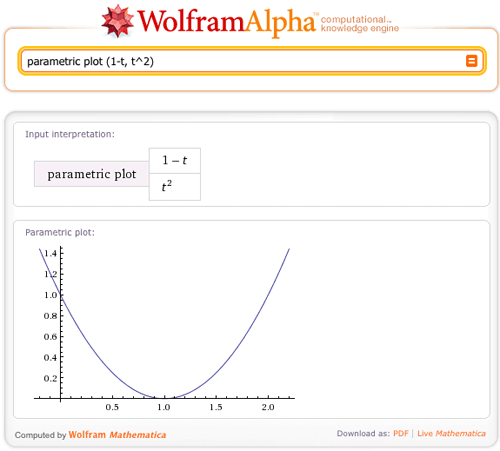We can easily see that this is the same as the Cartesian equation y = 1 – 2x + x2. (x(t) = 1 – tt = 1 – x(t) so y(x(t)) = (1 – x)2 = 1 – 2x + x2)

In the above example, we didn’t even need to enter a plot range; Wolfram|Alpha picked the plot range that best suits the graph. Of course, it’s possible to specify a range for the parameter, in this case we plot (x(t), y(t)) = (sin(t), sin(3t)) for t from 0 to 100.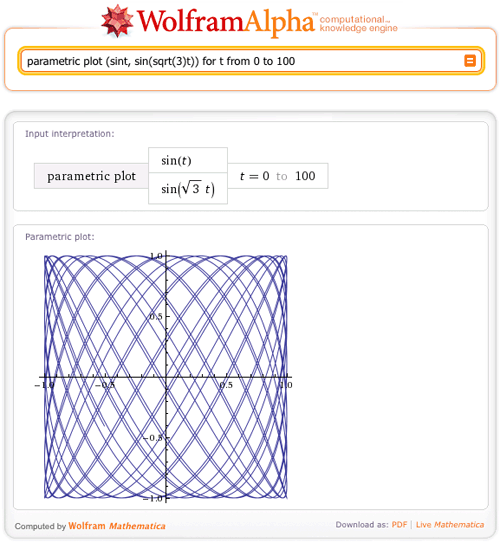Now let’s look at some other cool plots that Wolfram|Alpha can create.

How about the parametric plot of the astroid: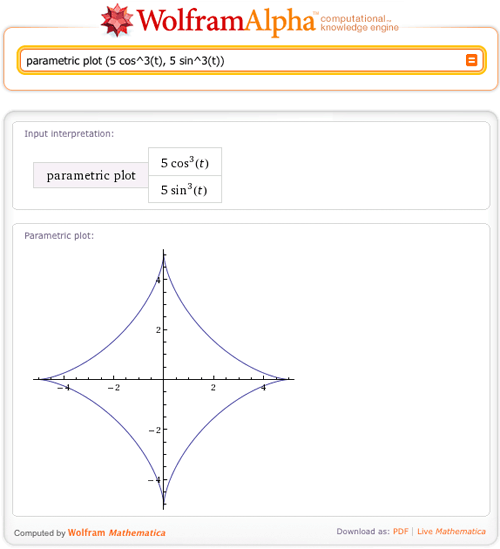Or a similar plot, the deltoid: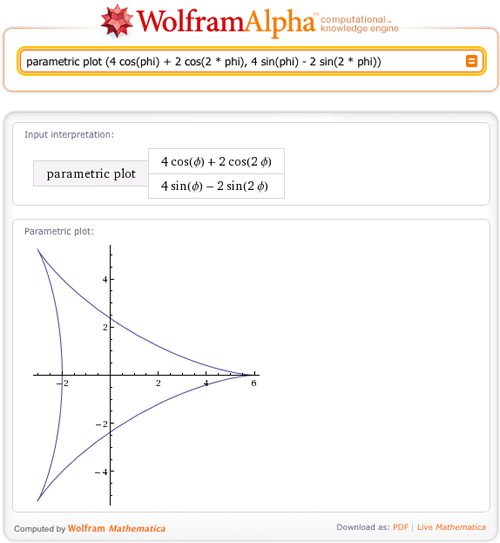If we had just said “plot” instead of “parametric plot”, then Wolfram|Alpha would have returned a Cartesian plot of 4cos(φ) + 2cos(2φ) and 4sin(φ) + 2sin(2φ), as well as a parametric plot of the deltoid.

Want some more examples? Check out these classic examples of parametric plots (the tractrix, fish curve, Tschirnhausen cubic, and Plateau curves, respectively):

In the previous plotting post, you had the opportunity to learn about 2D Cartesian plotting in Wolfram|Alpha, and now you are equipped with the ability to make 2D polar and parametric plots as well. Luckily, Wolfram|Alpha doesn’t stop there; start playing with some 3D graphs and it will not let you down!

Hello, can you tell me how to make plot with 2 function in it? so that I can see the intersection more clearly. Thank you very much.

Posted by Faldi Rianda May 15, 2012 at 9:32 pm

how to draw a curve like r=a(sin3?)where”?”is a range of angles 0°360°

Posted by jemima October 6, 2014 at 10:19 am

how to plot these point in polar coordinates
points are (-r,-0),(r,-0)
(0 mean “Theta”)

Posted by ruhma March 19, 2015 at 10:23 pm

Is there a way to include two plots on the same graph? I was hoping to show my students how to find the intersection of two polar curves, but wolfram alpha doesn’t seem to have the capacity to plot two functions on the same graph.

Thanks.

Posted by Dave Martin October 16, 2015 at 1:05 am